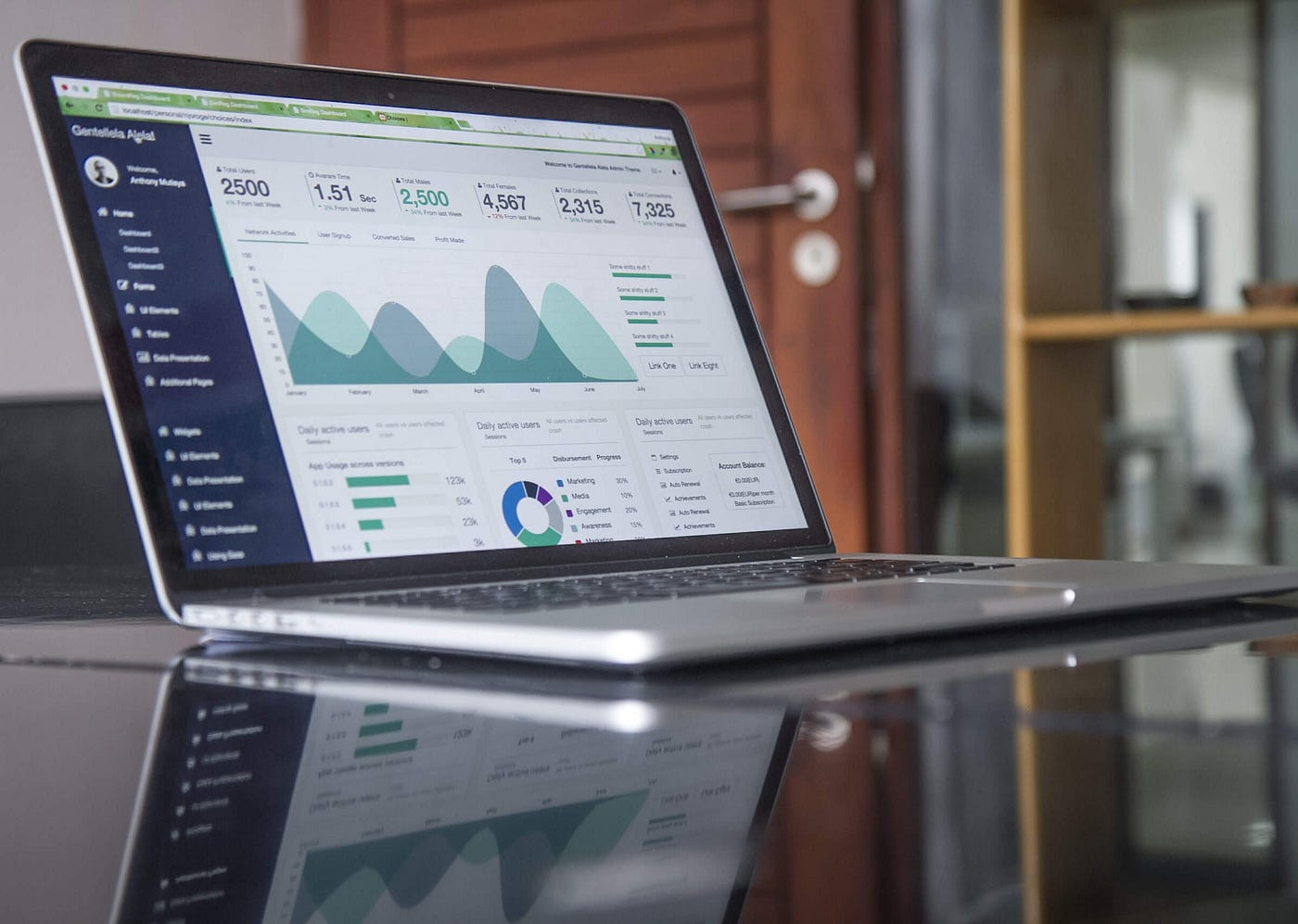# Learning Data Science: Day 8 - RegressionThe image is taken from Carlos Muza’s stock images.

After we spent our times on data exploration and data treatment, we are finally going to learn about regression. I’m pretty sure most of you have heard about regression. Since regression is usually the most popular techniques in predictive modeling, especially linear and logistic regressions.

# Definition

It is one of the predictive modeling techniques that correlate the relationship between the target value (dependent variable) with predictor values (independent variables). It is useful for forecasting, time series modeling, and finding the relationship between available variables.

Basically, what regression do is fitting the available data points to fit a curve/line to establish a model for future predictions.

# Benefits

There are two mains reasons for using regressions. Firstly, it shows how significant is the relationship between both dependent and independent variables. Secondly, it also helps to understand the level of impact between the multiple independent variables to the dependent variable. For example in the titanic dataset, we can analyze whether gender is related to the level of survival, and how strong the relation is.

# Types of regression

There are various types of regression, but here are the most common ones.

## 1. Linear Regression

Linear regression is one of the most popular ways to create a model and it is one of the regression technique that people start to learn predictive modeling. The constraint in linear regression is that the target value has to be continuous, meanwhile for the predictor values can be either continuous or discrete variable.

In establishing linear regression, a straight line will be used to indicate the best fit line to model the trend (also known as regression line). To obtain the regression line itself we use a technique called Least Square Method. The drawbacks of using linear regression are that there should be a linear relationship between the target and predictor values. It is also heavily affected by outliers.

## 2. Logistic Regression

When it comes to predicting whether events success or failure, logistic regression is the appropriate technique for that. It is mostly used for classification problems. One of logistic regression’s advantage is because it can handle various type of relationships, not limited to linear relationships. All significant variables should be included to avoid over-fitting and under-fitting.

## 3. Polynomial Regression

This kind of regression is similar to linear regression. However, the regression equation will be a polynomial. As the regression equation is polynomial, the regression line will be polynomial too. Be careful when trying to use a higher degree of the polynomial, since it may result in over-fitting. The tip is to observe the curve towards the ends.

## 4. Stepwise Regression

This regression can be used when we want to handle multiple independent variables. Stepwise regression is basically fits the model by adding/dropping co-variates one at a time based on certain criterion.

There are two types of selection in stepwise, forward and backward selection. Forward selection starts with the most significant independent variables then adding more variables for each step. Backward selection is the other way around where we start with all of the independent variables then remove the least significant variables for each step.

## Final Words

I know that it’s not covering all type of available regressions, but I do think for a started that’s the least we should know and understand. Do you have some regression technique that is worth to know? Put on the response below, or you can also create a discussion, I’ll be glad to discuss. Don’t hesitate if I have something that’s wrong in this story then just tell me. See you on the next story.

--

--